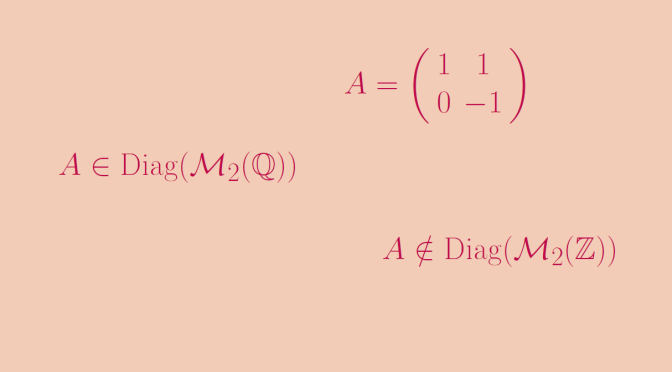# A diagonalizable matrix over Q but not over Z

Let us recall that a square matrix $$A$$ is called diagonalizable if it is similar to a diagonal matrix, i.e. if there exists an invertible matrix $$P$$ such that $$P^{-1}AP$$ is a diagonal matrix.

It is well known that a square matrix of dimension $$n \ge 1$$ over a field $$\mathbb{K}$$ that has $$n$$ distinct eigenvalues is diagonalizable. The proof is based on the fact that eigenvectors associated to different eigenvalues are linearly independent. Therefore a family of $$n$$ eigenvectors associated to the $$n$$ distinct eigenvalues is a basis. And over a field the matrix of the change basis from the standard basis to the basis of eigenvectors is invertible.

This doesn’t remain true if we consider a matrix over the ring of the integers $$\mathbb{Z}$$, even if the matrix is diagonalizable over the field $$\mathbb{Q}$$. For a counterexample we consider the matrix:
$A=\left(\begin{array}{cc} 1 & 1\\ 0 & -1\\ \end{array}\right)$ $$A$$ is an upper triangular matrix with distinct values on the main diagonal. Hence the elements of the main diagonal are eigenvalues. In the case of $$A$$, that means that $$1$$ and $$-1$$ are eigenvalues. As dimension of $$A$$ is equal to $$2$$, $$A$$ is diagonalizable on the field of the rational numbers $$\mathbb{Q}$$ according to above statement. More precisely, the matrix
$$P=\left(\begin{array}{cc} 1 & 1\\ 0 & -2\\ \end{array}\right)$$ is a matrix of eigenvectors, $$P^{-1}=\left(\begin{array}{cc} 1 & 1/2\\ 0 & -1/2\\ \end{array}\right)$$ and $$P^{-1}AP=\left(\begin{array}{cc} 1 & 0\\ 0 & -1\\ \end{array}\right)=D$$ is diagonal. However it is not possible to diagonalize $$A$$ over the ring $$\mathbb{Z}$$. If that was the case, an invertible matrix $$Q=\left(\begin{array}{cc} a & c\\ b & d\\ \end{array}\right)$$ would exist satisfying the equality $$AQ=QD$$.

The reader will verify that it forces $$Q$$ to be written as $$Q=\left(\begin{array}{cc} a & c\\ 0 & -2c\\ \end{array}\right)$$ with $$a,c$$ integers. Hence $$\det(Q)=2ac$$ cannot be an invertible element of $$\mathbb{Z}$$ and $$Q$$ cannot be an invertible matrix. We can finally conclude that $$A$$ is not diagonalizable over $$\mathbb{Z}$$. However, we notice that $$A$$ has two eigenvectors on $$\mathbb{Z}$$ and that $$A$$ is invertible over the ring of the integers.